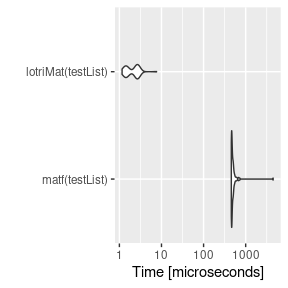# Motivation

This was made to allow people (like me) to specify lower triangular matrices similar to the domain specific language implemented in nlmixr. Originally I had it included in RxODE, but thought it may have more general applicability, so I separated it into a new package.

For me, specifying the matrices in this way is easier than specifying them using R’s default matrix. For instance to fully specify a simple 2x2 matrix, in R you specify:

mat <- matrix(c(1, 0.5, 0.5, 1),nrow=2,ncol=2,dimnames=list(c("a", "b"), c("a", "b")))

With lotri, you simply specify:

library(lotri)
library(microbenchmark)
library(ggplot2)

mat <- lotri(a+b ~ c(1,
0.5, 1))

I find it more legible and easier to specify, especially if you have a more complex matrix. For instance with the more complex matrix:

mat <- lotri({
a+b ~ c(1,
0.5, 1)
c ~ 1
d +e ~ c(1,
0.5, 1)
})

To fully specify this in base R you would need to use:

mat <- matrix(c(1, 0.5, 0, 0, 0,
0.5, 1, 0, 0, 0,
0, 0, 1, 0, 0,
0, 0, 0, 1, 0.5,
0, 0, 0, 0.5, 1),
nrow=5, ncol=5,
dimnames= list(c("a", "b", "c", "d", "e"), c("a", "b", "c", "d", "e")))

Of course with the excellent Matrix package this is a bit easier:

library(Matrix)
mat <- matrix(c(1, 0.5, 0.5, 1),nrow=2,ncol=2,dimnames=list(c("a", "b"), c("a", "b")))
mat <- bdiag(list(mat, matrix(1), mat))
## Convert back to standard matrix
mat <- as.matrix(mat)
##
dimnames(mat) <- list(c("a", "b", "c", "d", "e"), c("a", "b", "c", "d", "e"))

Regardless, I think lotri is a bit easier to use.

# Creating lists of matricies with attached properties

lotri also allows lists of matrices to be created by conditioning on an id with the | syntax.

For example:

mat <- lotri({
a+b ~ c(1,
0.5, 1) | id
c ~ 1 | occ
d +e ~ c(1,
0.5, 1) | id(lower=3, upper=2, omegaIsChol=FALSE)
})

print(mat)
#> $id #> d e #> d 1.0 0.5 #> e 0.5 1.0 #> #>$occ
#>   c
#> c 1
#>
#> Properties: lower, upper, omegaIsChol

print(mat$lower) #>$id
#> d e
#> 3 3
#>
#> $occ #> c #> -Inf print(mat$upper)
#> $id #> d e #> 2 2 #> #>$occ
#>   c
#> Inf
print(mat$omegaIsChol) #>$id
#>  FALSE

This gives a list of matrix(es) conditioned on the variable after the |. It also can add properties to each list that can be accessible after the list of matrices is returned, as shown in the above example. To do this, you simply have to enclose the properties after the conditional variable. That is et1 ~ id(lower=3).

## Combining symmetric named matrices

Now there is even a faster way to do a similar banded matrix concatenation with lotriMat

testList <- list(lotri({et2 + et3 + et4 ~ c(40,
0.1, 20,
0.1, 0.1, 30)}),
lotri(et5 ~ 6),
lotri(et1+et6 ~c(0.1, 0.01, 1)),
matrix(c(1L, 0L, 0L, 1L), 2, 2,
dimnames=list(c("et7", "et8"),
c("et7", "et8"))))

matf <- function(.mats){
.omega <- as.matrix(Matrix::bdiag(.mats))
.d <- unlist(lapply(seq_along(.mats),
function(x) {
dimnames(.mats[[x]])
}))
dimnames(.omega) <- list(.d, .d)
return(.omega)
}

print(matf(testList))
#>      et2  et3  et4 et5  et1  et6 et7 et8
#> et2 40.0  0.1  0.1   0 0.00 0.00   0   0
#> et3  0.1 20.0  0.1   0 0.00 0.00   0   0
#> et4  0.1  0.1 30.0   0 0.00 0.00   0   0
#> et5  0.0  0.0  0.0   6 0.00 0.00   0   0
#> et1  0.0  0.0  0.0   0 0.10 0.01   0   0
#> et6  0.0  0.0  0.0   0 0.01 1.00   0   0
#> et7  0.0  0.0  0.0   0 0.00 0.00   1   0
#> et8  0.0  0.0  0.0   0 0.00 0.00   0   1

print(lotriMat(testList))
#>      et2  et3  et4 et5  et1  et6 et7 et8
#> et2 40.0  0.1  0.1   0 0.00 0.00   0   0
#> et3  0.1 20.0  0.1   0 0.00 0.00   0   0
#> et4  0.1  0.1 30.0   0 0.00 0.00   0   0
#> et5  0.0  0.0  0.0   6 0.00 0.00   0   0
#> et1  0.0  0.0  0.0   0 0.10 0.01   0   0
#> et6  0.0  0.0  0.0   0 0.01 1.00   0   0
#> et7  0.0  0.0  0.0   0 0.00 0.00   1   0
#> et8  0.0  0.0  0.0   0 0.00 0.00   0   1

mb <- microbenchmark(matf(testList),lotriMat(testList))

print(mb)
#> Unit: microseconds
#>                expr     min       lq      mean   median       uq      max neval
#>      matf(testList) 455.769 462.7155 531.48916 472.4365 503.8590 4491.626   100
#>  lotriMat(testList)   1.159   1.4685   2.16534   2.3675   2.7545    7.636   100
autoplot(mb)
#> Coordinate system already present. Adding new coordinate system, which will replace the existing one.